机器学习-数据科学库（HM）_第4节_pandas1389-李同学

pandas

为什么要学习pandas?

• numpy帮助我们处理数值数据
• pandas帮我们处理数值数据+其它类型的数据

什么是pandas？

• pandas is an open source, BSD-licensed library providing high-performance, easy-to-use data structures and data analysis tools for the Python programming language.

pandas的常用数据类型

• series一维，带标签数组
• dataframe二维，series容器

pandas之Series创建

• series元素和index的数量必须一致
• pd.Series()：可以传入列表，也可以传入字典
1. t = pd.Series([1,2,3,4,5], index=list(“abcde”)
2. temp_dict = [“name”:“xiaohong”, “age”:30, “tel”:10086]
t = pd.Series(temp_dict )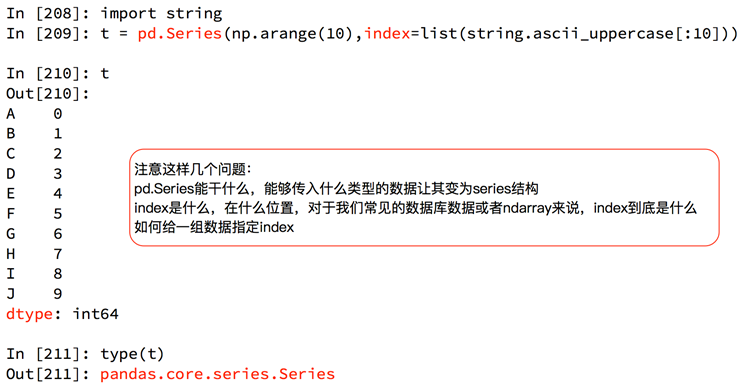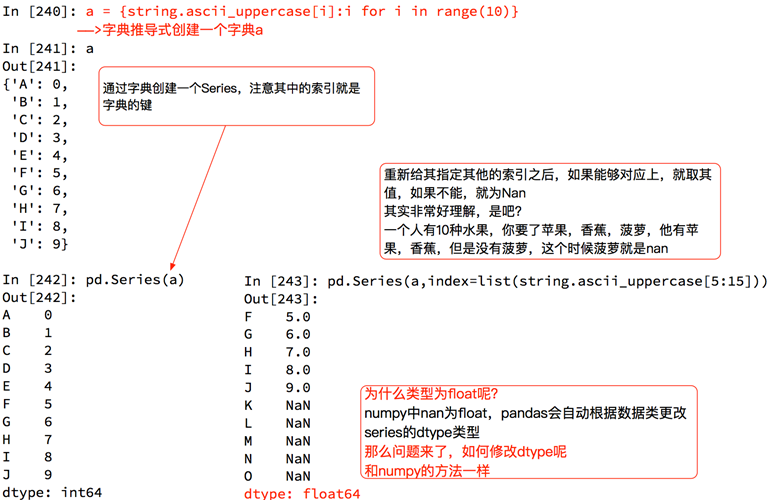pandas的切片和索引

• 切片：直接传入start，end，步长
• 索引：一个的时候直接传入序号或者index，多个的时候传入序号或者index的列表pandas之Series的索引和值

• Series对象本质上由两个数组构成
• index：索引（pandas.core.indexes.base.Index）
• values：值（numpy.ndarray）
• ndarray的很多方法都可以运用于series类型，比如aragmax，clip。
• series具有where方法，但结果和ndarray不同，具体可以查看官网。
• 可以看一下cookbook。
• t.index
• len(t.index)
• for i in t3.index: print(i)：打印所有index的值
• list(t3.index)[:2]：把index的前两位储存成list
• t.values

pandas之读取外部数据

• 数据类型是dataframe

pandas之Dataframe

• DataFrame对象既有行索引，又有列索引
• 行索引，表明不同行，横向索引，叫index，0轴，axis=0
• 列索引，表名不同列，纵向索引，叫columns，1轴，axis=1• 筛选dataframe读取的数据内容：• dataframe其他方法：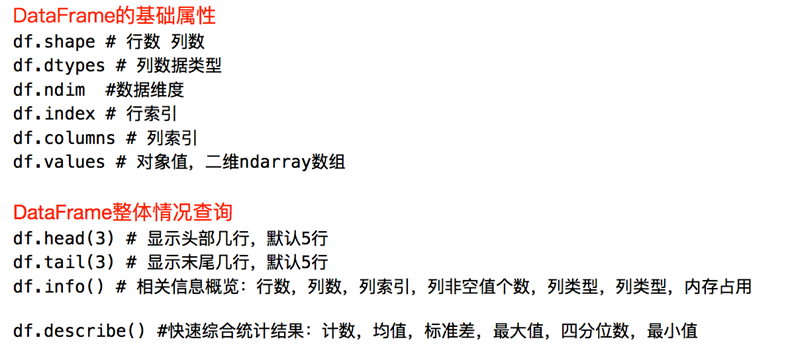– df.sort_values(by=“AnimalName”,ascending=False)
• by=“列的名称”，根据哪一列进行排序
• ascending=False，从大到小排序
• ascending=True，从小到大排序
• df[“Director”].unique()：“Director”这一列中导演的数量（不计算重复出现的导演）
• 计算演员的人数，但演员列中演员有多个，用逗号分割
• temp_actors_list = df[“Actors”].str.split(",").tolist()
• actors_list = [i for j in remp_actors_list for i in j]
• actors_sum = len(set(actors_list))
• argmin() / argmax()：返回最小值、最大值的index

pandas的取行取列

• df[“xxxx”]、df[:100][“colname”]
• 写字符串取列
• 方括号写数组（不能是单个数字），取行
• loc：通过标签索引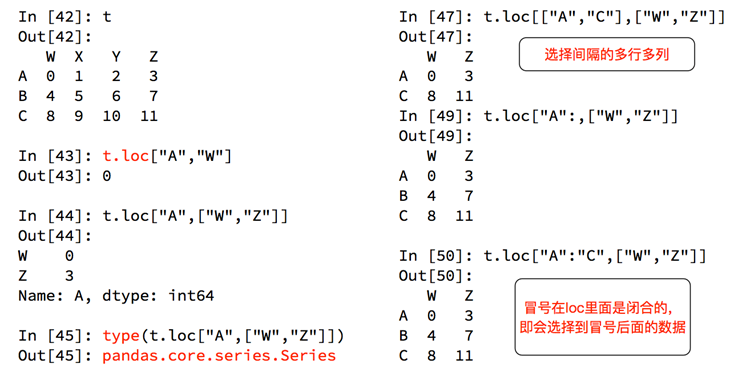• 取一行：t.loc[“A”] 、t.loc[“A”, :]
• 取一列：t.loc[:, “X”]
• 在loc里面冒号是闭合的
• star:end中如果end超出index范围，不报错
• iloc：通过位置索引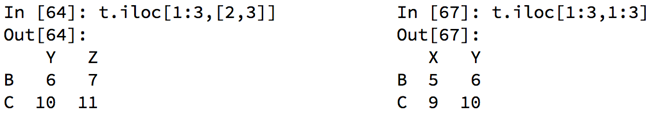pandas之布尔索引

• df[(800<df[“Count_AnimalName”]) & (df[“Count_AnimalName”]<1000)]：索引出Count_AnimalName这一行所有大于800小于1000的行
• df[(df[“Row_Labels”].str.len()>4) & (df[“Count_AnimalName”]>700)]：索引出次数超过700，并且名字的字符串的长度大于4的行
• & 和
• | 或

pandas之字符串方法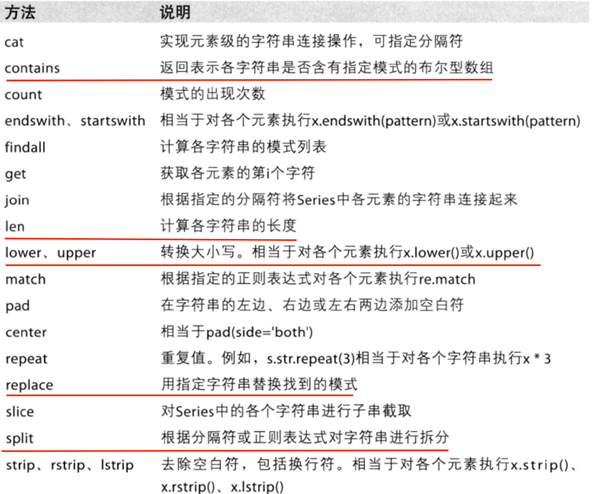• df[“info”].str.split("/").tolist()：把用“/”分割的df中的一列，并把它们返回为list

缺失数据的处理

• 缺失数据有两种情况
• 空、None、NaN
• 0
• 处理流程
• 判断为0的数据的意义：
• 如果是有实际意义的0则不做处理
• 如果是错误数据，则将它转换为nan：
• t[t==0]=np.nan
• 计算平均值等情况，nan不参与计算，但是0参与计算。
• 判断数据是否为NaN：pd.isnull(df),pd.notnull(df)
• 处理方式1：删除NaN所在的行列
• dropna(axis=0, how=‘any’, inplace=False)
• how=‘all’：该行中element全部为nan才删除
• how=‘any’：该行中element只要有一个为nan就删除
• inplace：是否原地替换
• 处理方式2：选取没有nan的行
• t3[pd.notnull(t3[“有nan的那一列”])]
• 处理方式3：填充数据
• t.fillna(t.mean()),t.fiallna(t.median()),t.fillna(0)
• 只用该列的中位数填充这一列：t[“age”] = t[“age”].fillna(t[“age”].mean())

评论 抢沙发觉得文章有用就打赏一下文章作者

支付宝扫一扫打赏微信扫一扫打赏Vieu3.3主题Q Q 登 录